lcheng

•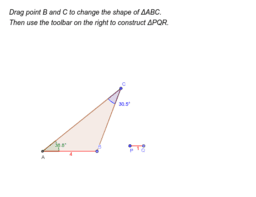Condition for Congruent Triangles 4 (AAS)

Activity

lcheng

•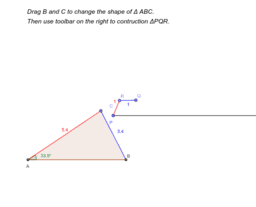SSA cannot be condition for congruent triangles

Activity

lcheng

•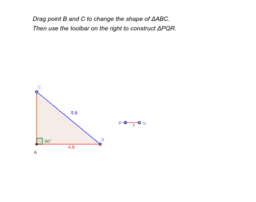Condition for Congruent Triangles 5 (RHS)

Activity

lcheng

•Condition for Congruent Triangles 4a (AAS)

Activity

lcheng

•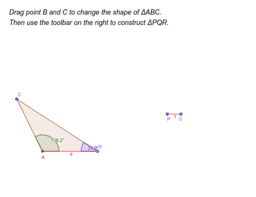Condition for Congruent Triangles 4 (ASA)

Activity

lcheng

•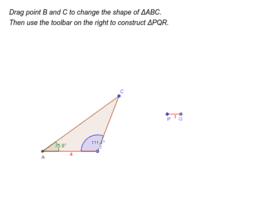Condition for Congruent Triangles 3 (ASA)

Activity

lcheng

•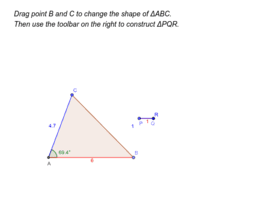Condition for Congruent Triangles 2 (SAS)

Activity

lcheng

•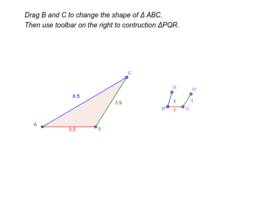Conditions for Congruent Triangles 1 (SSS)

Activity

lcheng

•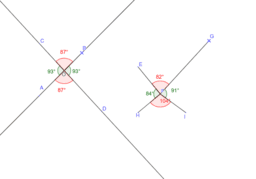Vertically Opposite Angles

Activity

lcheng

•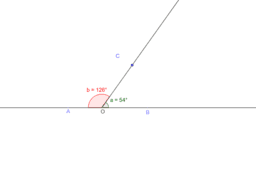Activity

lcheng

•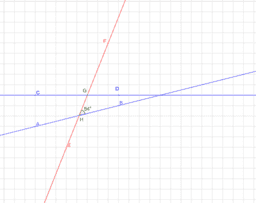Angles of Parallel Lines

Activity

lcheng

•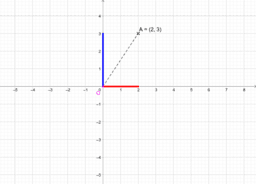Transformation 3 (Rotation)

Activity

lcheng

•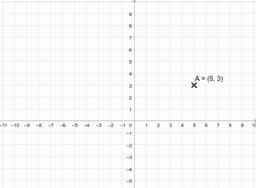Transformation 2 (Reflection)

Activity

lcheng

•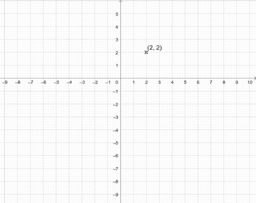Transformation 1 (Translation)

Activity

lcheng

•DSE2019Q19

Activity

lcheng

•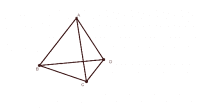Regular Tetrahedron

Activity

lcheng

•Sunrays with different angles

Activity

lcheng

•Right Pyramids

Activity

lcheng

•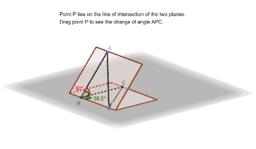Angle between Two Planes (Basic)

Activity

lcheng

•Pyramid with square base (angle between planes)

Activity

lcheng

•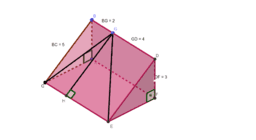Inclined plane (Angle between two planes)

Activity

lcheng

•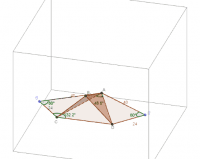DSE15 Q19 Example

Activity

lcheng

•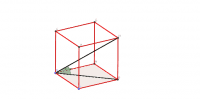Cube (Angle between a line and a plane)

Activity

lcheng

•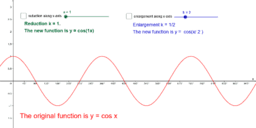Activity

lcheng